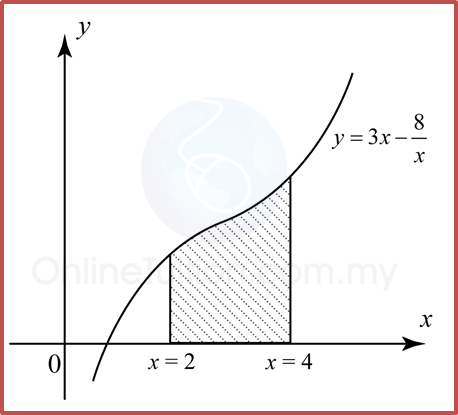# 3.6 Integration as the Summation of Volumes

3.6 Integration as the Summation of Volumes

(1).The volume of the solid generated when the region enclosed by the curve y = f(x), the x-axis, the line x = and the line x = b is revolved through 360° about the x-axis is given by

${V}_{x}=\pi {\int }_{a}^{b}{y}^{2}dx$

(2).The volume of the solid generated when the region enclosed by the curve x = f(y), the y-axis, the line y = a and the line y = b is revolved through 360° about the y-axis is given by
${V}_{y}=\pi {\int }_{a}^{b}{x}^{2}dy$

Example 1:
Find the volume generated for the following diagram when the shaded region is revolved through 360° about the x-axis.Solution:
Volume generated, Vx
$\begin{array}{l}{V}_{x}=\pi {\int }_{a}^{b}{y}^{2}dx\\ {V}_{x}=\pi {\int }_{2}^{4}{\left(3x-\frac{8}{x}\right)}^{2}dx\\ {V}_{x}=\pi {\int }_{2}^{4}\left(3x-\frac{8}{x}\right)\left(3x-\frac{8}{x}\right)dx\\ {V}_{x}=\pi {\int }_{2}^{4}\left(9{x}^{2}-48+\frac{64}{{x}^{2}}\right)dx\\ {V}_{x}=\pi {\left[\frac{9{x}^{3}}{3}-48x+\frac{64{x}^{-1}}{-1}\right]}_{2}^{4}\\ {V}_{x}=\pi {\left[3{x}^{3}-48x-\frac{64}{x}\right]}_{2}^{4}\\ {V}_{x}=\pi \left[\left(3{\left(4\right)}^{3}-48\left(4\right)-\frac{64}{4}\right)-\left(3{\left(2\right)}^{3}-48\left(2\right)-\frac{64}{2}\right)\right]\\ {V}_{x}=\pi \left(-16+104\right)\\ {V}_{x}=88\pi \text{}uni{t}^{3}\end{array}$

Example 2:
Find the volume generated for the following diagram when the shaded region is revolved through 360° about the y-axis.Solution:
Volume generated, Vy
$\begin{array}{l}{V}_{y}=\pi {\int }_{a}^{b}{x}^{2}dy\\ {V}_{y}=\pi {\int }_{1}^{2}{\left(\frac{2}{y}\right)}^{2}dy\\ {V}_{y}=\pi {\int }_{1}^{2}\left(\frac{4}{{y}^{2}}\right)dy\\ {V}_{y}=\pi {\int }_{1}^{2}4{y}^{-2}dy\\ {V}_{y}=\pi {\left[\frac{4{y}^{-1}}{-1}\right]}_{1}^{2}=\pi {\left[-\frac{4}{y}\right]}_{1}^{2}\\ {V}_{y}=\pi \left[\left(-\frac{4}{2}\right)-\left(-\frac{4}{1}\right)\right]\\ {V}_{y}=2\pi \text{}uni{t}^{3}\end{array}$